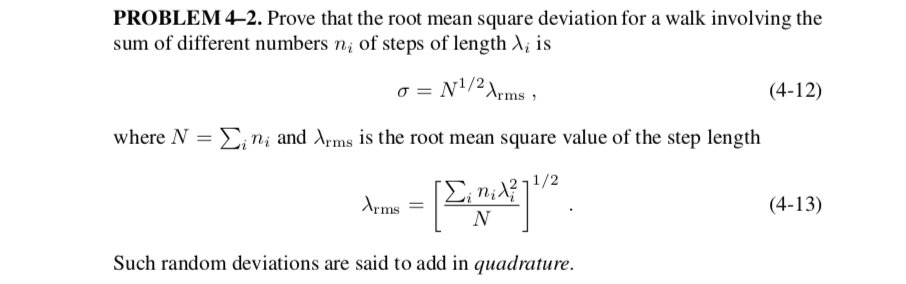# How to show the root mean square deviation

Homework Statement:
prove that the mean position after a given number of steps is the starting position
Relevant Equations:
random walkHi
I tried like this.
##σ^2=<(λ_1+λ_2+,,,+λ_i)^2>=<λ_1^2>+<λ_2^2>+,,,<λ_i^2>##
And I know ##σ^2=Σ_in_iλ_i^2##from equation (4-12) (so this is cheat).

So I know also ##<λ_i^2>=n_iλ_i^2##, But why??

I know if I take ##λ=1 ,σ^2=n##,But I don't understand ##λ≠1## version.

RPinPA
Homework Helper
I tried like this.
##σ^2=<(λ_1+λ_2+...+λ_i)^2>=<λ_1^2>+<λ_2^2>+...+<λ_i^2>##

Why do you think that is true? If I understand the problem correctly, the ##\lambda_i## are fixed parameters and so are the ##n_i##. You need a random variable here.

Are you studying random walks in one dimension? Then let's define a random variable ##\Lambda_{ij}##, ##j = 1,n_i## which is either ##\lambda_i## or ##-\lambda_i## with equal probability. That is, corresponding to each ##\lambda_i## (fixed) there are ##n_i## (fixed number) of steps, each of which is either ##\lambda_i## to the right or ##\lambda_i## to the left (randomly).

Let's start with a simpler example. Let's suppose all the steps have the same size ##\lambda_1## and there are ##n_1## of them. So ##N = n_1##. So we have ##\Lambda_{11}, \Lambda_{12}, ..., \Lambda_{1,n_1} = \pm\lambda_1##. Obviously each has zero mean.

The total square deviation we are interested in is then ##\sigma^2 = <\left ( \sum_{j=1}^{n_1} \Lambda_{1j} \right )^2 > = \sum_{j=1}^{n_1} < \Lambda_{1j}^2 >## since the ##\Lambda_{1j}## are independent and the expectation of the cross terms is 0. Thus ##\sigma^2## in this case reduces to ##n_1 <\Lambda_{11}^2>##.

This is the familiar result that ##\sigma^2## is proportional to the number of steps. The final thing here (I leave it to you) is to replace the expectation value of ##\Lambda_{11}^2## by something in terms of the fixed parameters ##\lambda##.

You may think that this is what you were doing, but it's not. You didn't have a random variable in your expression, and you didn't have the right number of terms. You need to have a random variable for each of the ##n_i## steps of each size, as I did even when there is only one step size. So your steps will be ##\Lambda_{11}, \Lambda_{12}, ..., \Lambda_{1n_1} = \pm\lambda_1## and ##\Lambda_{21}, \Lambda_{22}, ..., \Lambda_{2n_2} = \pm \lambda_2##, etc., and you will need double sums.

•arcTomato
At first, Thank you for reply. Your explanation is so easy to understand.
I think I didn't understand the problem correctly.

I have to take the length like##Λ_{i,1},Λ_{i,2}...,Λ_{i,n_i}=±λ_i##.
so
##\sigma^2 = <\left ( \sum_{j=1}^{n_i}\sum_{i} \Lambda_{ij} \right )^2 > = \sum_{i} \sum_{j=1}^{n_i} < \Lambda_{ij}^2 >=\sum_{i} n_i(±\lambda_{i})^2 ##
I think this is almost the answer. Right??

Last edited: